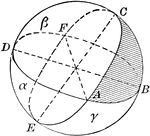### Area of Spherical Triangle

Diagram used to prove the theorem: "The area of a spherical triangle, expressed in spherical degrees,…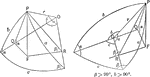### Relationships In A Spherical Triangle

Illustration used, with the law of sines, to find the relation between two sides of a spherical triangle…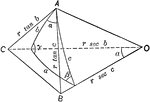### Relationships In A Spherical Triangle

Illustration used, with the law of cosines, to find the relation between the three sides and an angle…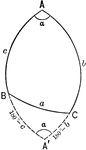### Relationships In A Spherical Triangle

Illustration used to extend the law of cosines when finding the relation between the three sides and…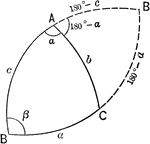### Relationships In A Spherical Triangle

Illustration used to extend the law of cosines when finding the relation between the three sides and…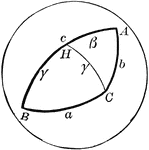### Side Comparison of Spherical Triangle

Diagram used to prove the theorem: "In a spherical triangle, the greater side is opposite the greater…### Symmetrical Spherical Triangles

Diagram showing 2 symmetrical spherical triangles.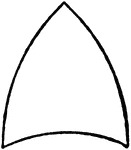### Spherical Triangle

Diagram showing a spherical triangle.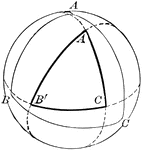### Spherical Triangle

Diagram showing a spherical triangle constructed by the intersection of polar arcs.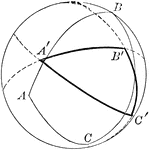### Spherical Triangle

Diagram showing a spherical triangle constructed by the intersection of polar arcs.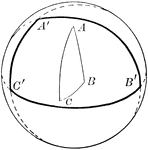### Spherical Triangle

Diagram showing a spherical triangle constructed by the intersection of polar arcs.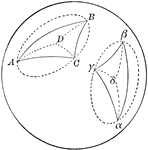### Two Symmetrical Spherical Triangles

Diagram showing a 2 symmetrical spherical triangles.